# I don't know how to get the x-y direction of position P

requied
Homework Statement:
Electric field at a plastic line in square shape
Relevant Equations:
E = kQ/r2
lambda = q/l
Please help me to find the position P and whether it will work in this solution by knowing the position.
(The question, my solution and thought in the image)

#### Attachments

•çözümlü.png
37.7 KB · Views: 125

Mentor
Homework Statement:: Electric field at a plastic line in square shape
Relevant Equations:: E = kQ/r2
lambda = q/l

Please help me to find the position P and whether it will work in this solution by knowing the position.
(The question, my solution and thought in the image)
Does this help? You can add up the vector contributions of the 4 line charges:

http://hyperphysics.phy-astr.gsu.edu/hbase/electric/elelin.html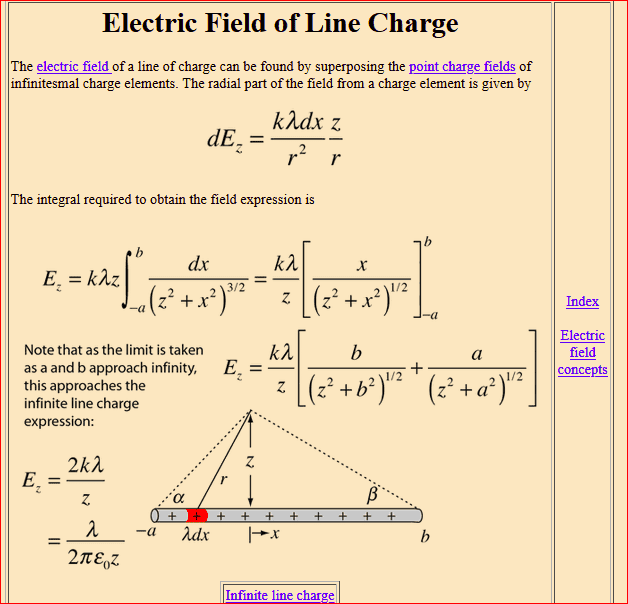•requied
requied
Thank you so much!

•berkeman
requied
Last edited by a moderator:
Homework Helper
Gold Member
How about showing an attempt at the solution? First draw the point of interest inside the square. Then use the result from post #2 to find an expression for one of the line segments. Be sure to relate correctly ##a##, ##b## and ##z## in the link to ##x_p##, ##y_p## and ##a## in the drawing you are given. Once you have figured this out, the other three segments should be done the same way. I very strongly suggest that you use unit vector notation to express all vectors, because in the end you will have to add all four contributions together, i.e. superimpose them; unit vectors make superposition much much easier.

•etotheipi and berkeman
requied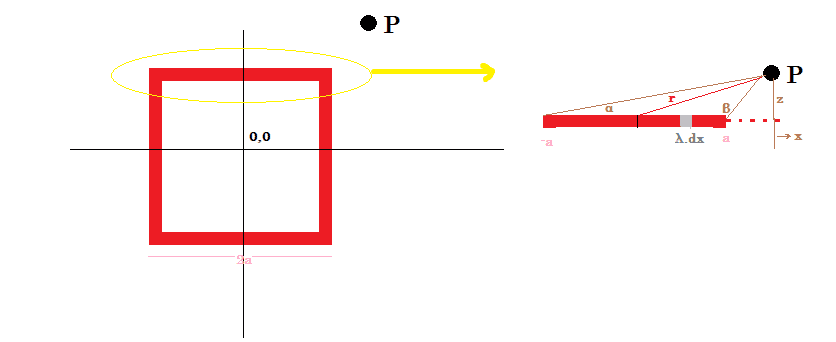what if I do that by superposing thing? Could it give the same result? Also I don't actually know what E_z is.

Homework Helper
Gold Member
View attachment 261187
what if I do that by superposing thing? Could it give the same result? Also I don't actually know what E_z is.
I don't understand what you mean by "do that by superposing thing". ##E_z## is the electric field at point P in the hyperphysics drawing. It points from the bottom of the screen towards the top. There is also a component in the ##x## direction for which you have to find an additional formula similar to that shown in the hyperphysics link.

It helps to draw a complete drawing such as the one I drew when I did this problem (see below). Note that I use ##x## and ##y## axes instead of ##x## and ##z##. Start by finding an expression for ##E_y## for the bottom wire segment using the hyperphysics formula. Then derive another formula for ##E_x##. Finally, adapt the two formulas to the remaining 3 sides. It's a lot of work but it's also straightforward.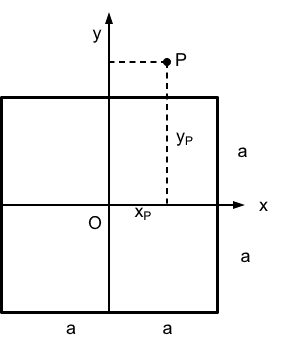•requied
requied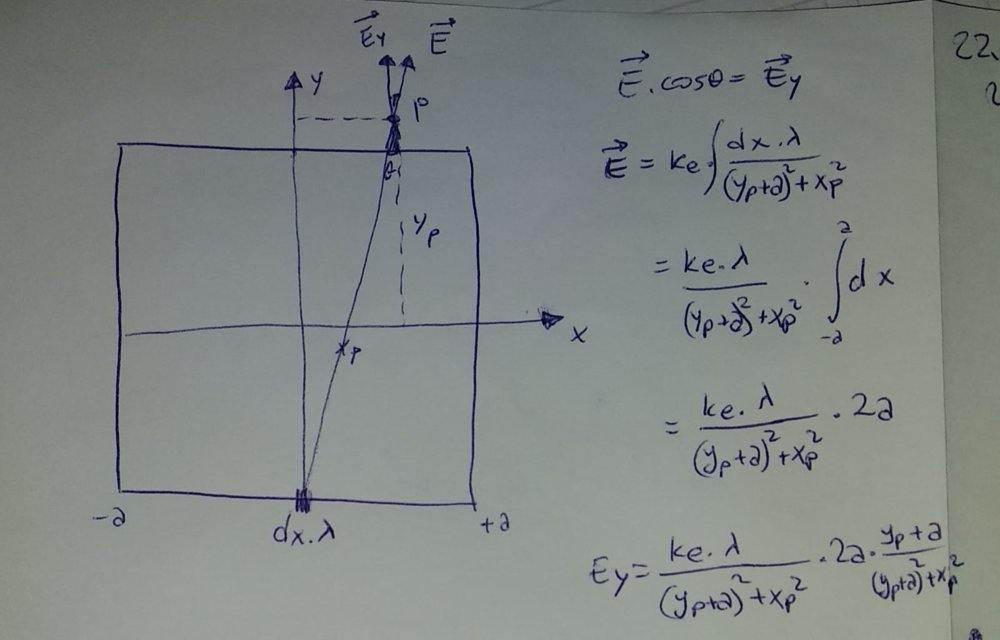How can I get rid of these Xp and yp.So would they stay or should cancel each other? And what did you mean by saying that 'derive another formula for Ex'?

Editing: I noticed that I had made a lot of mistake at finding the expression of Ey. I hope this one has less mistakes. Would you check it whether I did correct or wrong.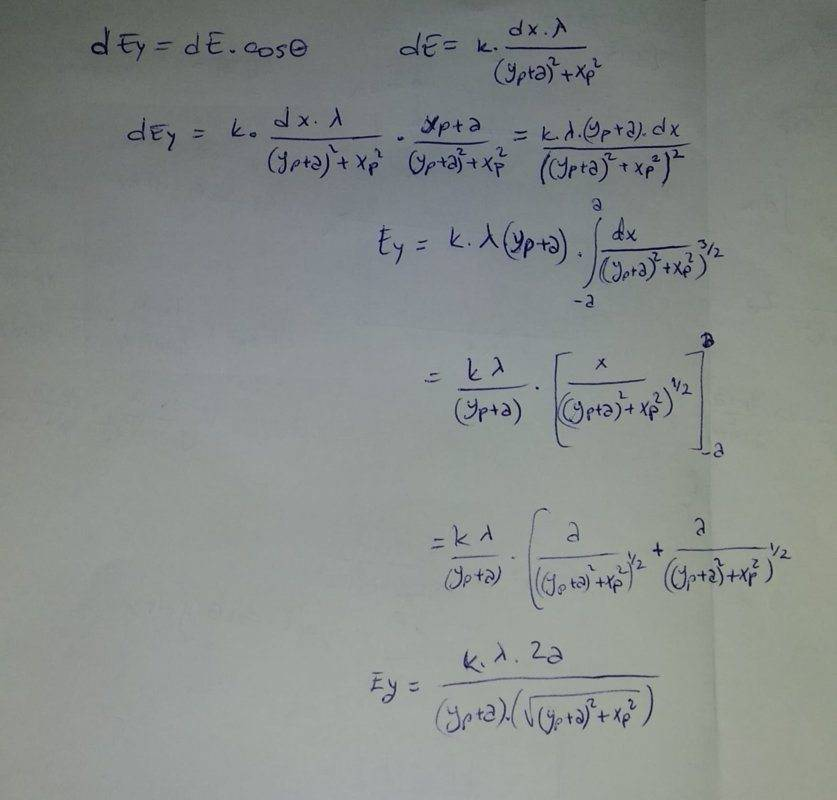Last edited:
Homework Helper
Gold Member
You cannot get rid of ##x_p## and ##y_p##. They give you the coordinates where you have to calculate the field relative to the center of the square marked by O in my drawing. Look at it. The electric field at point P has two components, one (##E_y##) is straight up because all the charge (assumed to be positive) is below it and the other one (##E_x##) is to the right because there is more charge to the left of P than to the right. It is that component that you have to find a formula for.

Your integral is set up incorrectly. You cannot take the source point on the y-axis at x = 0 but at an arbitrary non-zero point ##x##. Then the distance from the element to P would be ##r=\left[(x_p-x)^2+(a+y_p)^2\right]^{1/2}##. Next you need to write the magnitude of element ##dE##, find its x and y components and integrate those.

•requied
Homework Helper
Gold Member
2022 Award
There is something strange in the problem statement. Why xp>a√2 and yp>a√2? The diagram looks more like xp<a and yp>a.

•requied
Homework Helper
Gold Member
There is something strange in the problem statement. Why xp>a√2 and yp>a√2? The diagram looks more like xp<a and yp>a.
Yes, point P should be more correctly drawn outside the circle that circumscribes the square.

•requied
requied
You cannot get rid of ##x_p## and ##y_p##. They give you the coordinates where you have to calculate the field relative to the center of the square marked by O in my drawing. Look at it. The electric field at point P has two components, one (##E_y##) is straight up because all the charge (assumed to be positive) is below it and the other one (##E_x##) is to the right because there is more charge to the left of P than to the right. It is that component that you have to find a formula for.

Your integral is set up incorrectly. You cannot take the source point on the y-axis at x = 0 but at an arbitrary non-zero point ##x##. Then the distance from the element to P would be ##r=\left[(x_p-x)^2+(a+y_p)^2\right]^{1/2}##. Next you need to write the magnitude of element ##dE##, find its x and y components and integrate those.
Where does come x you have mentioned as 'Then the distance from the element to P would be r=[(xp−x)2+(a+yp)2]1/2 '

first edit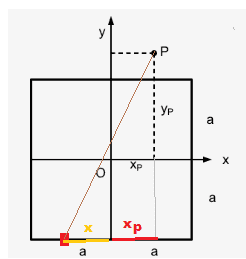Might you mean 'xp + x' ?

second edit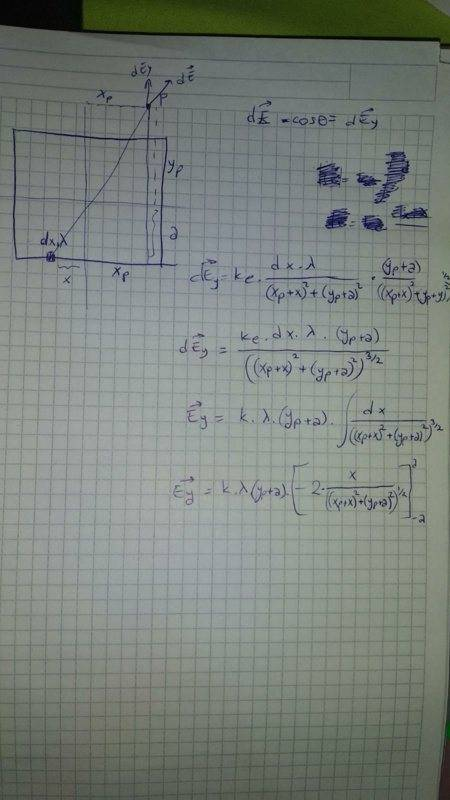Is there any problem in sight that you saw?

Last edited:
Homework Helper
Gold Member
2022 Award
Yes, point P should be more correctly drawn outside the circle that circumscribes the square.
No, the text says it should be within the quadrant up and to the right of the point (a√2, a√2). That is well outside the square, and there is no apparent reason for such a constraint.
Seems to me more likely the diagram is right and the text wrong. The constraint should be 0<xp<a, a<yp.

•requied
Homework Helper
Gold Member
No, the text says it should be within the quadrant up and to the right of the point (a√2, a√2). That is well outside the square, and there is no apparent reason for such a constraint.
Seems to me more likely the diagram is right and the text wrong. The constraint should be 0<xp<a, a<yp.
I would agree to go with the diagram and ignore the text. One reason I can think for the constraint is one of convenience for the author of the problem who will have to evaluate the answers. The expressions for the field components are tediously involved and would be difficult to decide whether an answer is correct if point P is not in the same general area as the the author's solution.

•requied
Homework Helper
Gold Member
2022 Award
View attachment 261214
Is there any problem in sight that you saw?
You seem to have taken x as measured leftwards from the origin. It would be safer to take it in the usual rightward direction, giving xp-x as the displacement. What you got may have a sign error.

Other than that, you were doing well until the integration. It's a good idea to check dimensionality occasionally. The integrand had dimension L-2, but the result you got is dimensionless.
And where did the 2 come from?

Check again that you have applied the formula from post #2 correctly. Note that you have x+xp where the formula has x, so a shift in range is needed. And look at what happened to the z in front of the integral.

If you can't find your mistake, differentiate the integration result you got and see if it gets back to the integrand.

Last edited:
•requied
requied
My integration skill is not enough to take, I just looked at some integral calculators to figure out for steps but since I saw arctanx, secx etc. I guess I will leave from this question. Thank you all for answers and attention.

Homework Helper
Gold Member
2022 Award
My integration skill is not enough to take, I just looked at some integral calculators to figure out for steps but since I saw arctanx, secx etc. I guess I will leave from this question. Thank you all for answers and attention.
You don't need to do the integration yourself if you apply the equation at the link correctly.
It starts with ##\int_{x=-A}^B\frac {z.dx}{(z^2+x^2)^\frac 32}##.
(I changed a, b to uppercase to avoid confusion with your a.)
You have to make it look like your ##\int_{x=-a}^a\frac {(y_p+a).dx}{((y_p+a)^2+(x_p+x)^2)^\frac 32}##
Their z corresponds to your yp+a. You substituted that correctly inside the denominator but in the other place you kept it in the numerator instead of moving it to the denominator.
Their x corresponds to your xp+x. So you must replace every x in the text at the link with xp+x: ##\int_{x+x_p=-A}^{x+x_p=B}\frac {z.d(x+x_p)}{(z^2+(x+x_p)^2)^\frac 32}##.
Note what has happened to the bounds. To make them like your bounds we need A=a-xp and B=a+xp. So use those values in their answer.

Homework Helper
Gold Member
I agree with @haruspex that you don't need to do the integral for the y-component if you apply the link correctly. However you still need to do the integral for the x-component because the link does not provide that. Here is how you set up both integrands.

The magnitude of the contribution to the field from element ##\lambda dx## is
##dE=\dfrac{k\lambda dx}{(x+x_p)^2+(a+y_p)^2)}##
Let ##\theta## be the angle between that x-axis and the vector from the charge element ##\lambda dx## to point P.
The x-component of ##dE## is
##dE_x=dE \cos\theta=\dfrac{k\lambda (x+x_p) dx}{\left[(x+x_p)^2+(a+y_p)^2)\right]^{3/2}}##
The y-component of ##dE## is
##dE_y=dE \sin\theta=\dfrac{k\lambda (a+y_p) dx}{\left[(x+x_p)^2+(a+y_p)^2)\right]^{3/2}}##
The integration limits are from ##x=-a## to ##x=+a##. These can be reduced by substitution to the basic forms$$I_1=\int\frac{du}{(u^2+c^2)^{3/2}}~;~~I_2=\int\frac{udu}{(u^2+c^2)^{3/2}}$$If you cannot do them by yourself, look them up on the web.

requied
I was studying on other lectures. So I wasn't here for a month. I just glanced all comments above and I decided to choose a point on the upper line this time.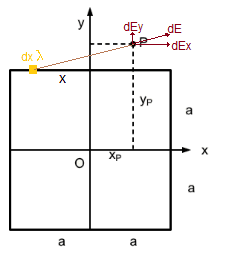##dE_y=dE \sin\theta= \ k\ y_p\lambda∫ \dfrac{ dx}{\left[(x+x_p)^2+(y_p-a)^2)\right]^{3/2}}##

##dE_y= \ k\ y_p\lambda∫ \dfrac{ dx}{\left[(x+x_p)^2+\left[(x+x_p)^2tan^2\ x)\right]\right]^{3/2}}## ## --->## ##tan\theta\ =\ y_p-a\ /\ x+x_p ##

##dE_y= \ k\ y_p\lambda∫ \dfrac{ dx}{\left[(x+x_p)^2*\left[(1+tan^2\ x)\right]\right]^{3/2}}##

If I get over this integration, the question will be over. This is the point we have reached so far. Since ##y_p -a## will not change, I tried to write it as ##x_p## but I guess it didn't work. What should I do now?

Homework Helper
Gold Member
2022 Award
##dE_y=dE \sin\theta= \ k\ y_p\lambda∫ \dfrac{ dx}{\left[(x+x_p)^2+(y_p-a)^2)\right]^{3/2}}##

##dE_y= \ k\ y_p\lambda∫ \dfrac{ dx}{\left[(x+x_p)^2+\left[(x+x_p)^2tan^2\ x)\right]\right]^{3/2}}## ## --->## ##tan\theta\ =\ y_p-a\ /\ x+x_p ##
Sorry, but this is quite wrong.
To do a substitution you need to express it in the form x=... then use that to substitute for every occurrence of x. That includes inside 'dx' and in the range bounds.
Also, outside the integral you should have (yp-a).

Anyway, as I posted, you do not really need to do the integration yourself. You can use the standard result, but you do have to make the correct match up between your variables and the ones at the link. I can help you with that, but I'm busy for the next several hours.

Last edited:
Homework Helper
Gold Member
2022 Award
Ok, I'm back...
Your expression is (or should be) ##k\lambda (y_p-a)\int_{x=-a}^{x=a}\frac{dx}{((x+x_p)^2+(y_p-a)^2)^{\frac 32}}##
To avoid confusion, I'll rewrite the integral at the linked page as ##k\lambda z\int_{t=-A}^{t=B}\frac{dt}{(z^2+t^2)^{\frac 32}}##.
To match this up to your integral :
##z=y_p-a##
##t=x+x_p##
Plug those in, then work out what you have to replace A and B by to complete the conversion.

requied
Can't we solve this by not touching on matching ##k\lambda (y_p-a)\int_{x=-a}^{x=a}\frac{dx}{((x+x_p)^2+(y_p-a)^2)^{\frac 32}}## and ##k\lambda z\int_{t=-A}^{t=B}\frac{dt}{(z^2+t^2)^{\frac 32}}##. Because I couldn't understand replacing A and B in the linked page somehow. I mean isn't there any possibility to solve ##k\lambda (y_p-a)\int_{x=-a}^{x=a}\frac{dx}{((x+x_p)^2+(y_p-a)^2)^{\frac 32}}## as it is?

Ok, I'm back...
Your expression is (or should be) ##k\lambda (y_p-a)\int_{x=-a}^{x=a}\frac{dx}{((x+x_p)^2+(y_p-a)^2)^{\frac 32}}##
To avoid confusion, I'll rewrite the integral at the linked page as ##k\lambda z\int_{t=-A}^{t=B}\frac{dt}{(z^2+t^2)^{\frac 32}}##.
To match this up to your integral :
##z=y_p-a##
##t=x+x_p##
Plug those in, then work out what you have to replace A and B by to complete the conversion.

Homework Helper
Gold Member
2022 Award
Can't we solve this by not touching on matching ##k\lambda (y_p-a)\int_{x=-a}^{x=a}\frac{dx}{((x+x_p)^2+(y_p-a)^2)^{\frac 32}}## and ##k\lambda z\int_{t=-A}^{t=B}\frac{dt}{(z^2+t^2)^{\frac 32}}##. Because I couldn't understand replacing A and B in the linked page somehow. I mean isn't there any possibility to solve ##k\lambda (y_p-a)\int_{x=-a}^{x=a}\frac{dx}{((x+x_p)^2+(y_p-a)^2)^{\frac 32}}## as it is?
Of course, and I described how to do that in post #20:
"To do a substitution you need to express it in the form x=... then use that to substitute for every occurrence of x. That includes inside 'dx' and in the range bounds."

requied
##\int_{x=-a}^a\frac {(y_p-a).dx}{((y_p-a)^2+(x_p+x)^2)^\frac 32}##

##k\ lambda\ (y_p-a)\int_{a-x_p=-A}^{a+x_p=B}\frac {d(x+x_p)}{((y_p-a)^2+(x+x_p)^2)^\frac 32} =\frac {(x_p+x)}{[(x_p+x)^2+(y_p-a)^2]^\frac{1}{2}} ## with borders from ##a-x_p## to ##a+x_p##

Is this right?
Note: I cheated little bit from your #17th post :)

Homework Helper
Gold Member
2022 Award
##\int_{x=-a}^a\frac {(y_p-a).dx}{((y_p-a)^2+(x_p+x)^2)^\frac 32}##

##k\ lambda\ (y_p-a)\int_{a-x_p=-A}^{a+x_p=B}\frac {d(x+x_p)}{((y_p-a)^2+(x+x_p)^2)^\frac 32} =\frac {(x_p+x)}{[(x_p+x)^2+(y_p-a)^2]^\frac{1}{2}} ## with borders from ##a-x_p## to ##a+x_p##

Is this right?
Note: I cheated little bit from your #17th post :)
Going from ##\int\frac {(y_p-a).dx}{((y_p-a)^2+(x_p+x)^2)^\frac 32}## to ##\ (y_p-a)\int\frac {d(x+x_p)}{((y_p-a)^2+(x+x_p)^2)^\frac 32}## did not involve a substitution, so the bounds should not change. You should have ##\ (y_p-a)\int_{x=-a}^{x=a}\frac {d(x+x_p)}{((y_p-a)^2+(x+x_p)^2)^\frac 32}##.
A bound like ##a+x_p=B## is meaningless. If the integration variable is x then a bound should specify a value of x: "x=..."

Homework Helper
Gold Member
Would you give more details about 'superposing' ?
I think you mean 'superimpose'. That means that you can solve the problem in simple parts (like one side at a time) as though the other parts are not there, and then sum up the partial answers for the final total answer.

Homework Helper
Gold Member
2022 Award
I think you mean 'superimpose'
I think either is ok. A superposition of states, e.g.

•FactChecker
requied
The final solution is this then:

##\frac{k\ lambda}{(y_p-a)}\ =\frac {(x_p+x)}{[(x_p+x)^2+(y_p-a)^2]^\frac{1}{2}} ## with borders from ##-a## to ##a##

I don't know where ##(y_p-a)## in denominator came from in the linked page at #2. In link ##z## = ##(y_p-a)##
now. Please tell me that is true because it became boring for me :/